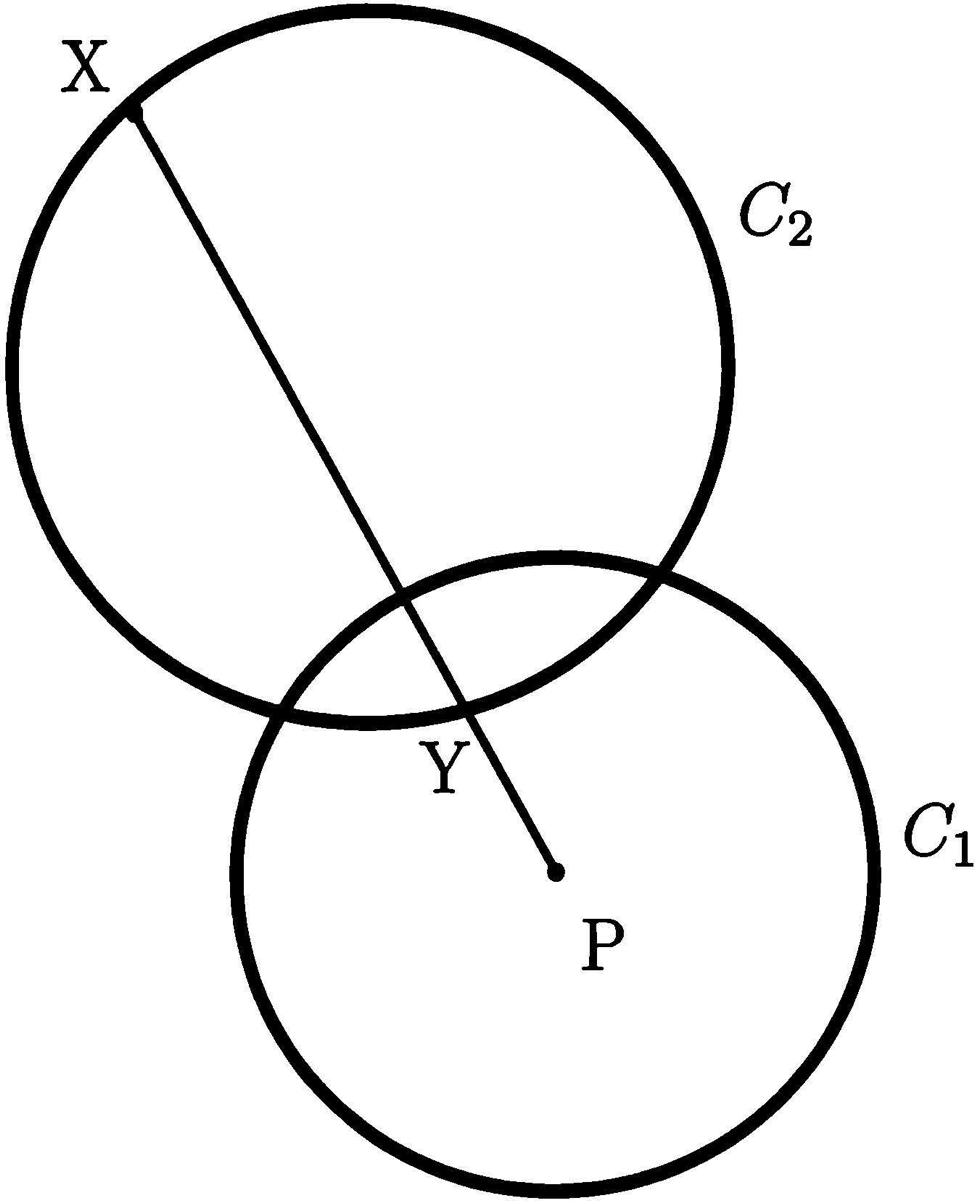# Big Picard theorem

Originally published at 狗和留美者不得入内. You can comment here or there.

I’ve been asked to prove the Big Picard theorem, assuming the fundamental normality test. Assuming the latter, it is a very short proof, and I could half-ass with that. I don’t like writing up stuff that I don’t actually understand for the sake of doing so. There’s little point, and if I’m going to actually write up a proof of it, I’ll do so for real, which means that I go over the fundamental normality test in its entirety.

First some preliminaries.

Theorem 2.28 (Riemann mapping theorem). Let$\Omega \subset \mathbb{C}$ be simply-connected and$\Omega \neq \mathbb{C}$. Then there exists a conformal homeomorphism$f : \Omega \to \mathbb{D}$ onto the unit disk$\mathbb{D}$.

Theorem 2.30. Suppose n is bounded, simply-connected, and regular. Then any conformal homeomorphism as in Theorem 2.28 extends to a homeomorphism$\bar{\Omega} \to \bar{\mathbb{D}}$.

Schwarz reflection principle. Suppose that$f$ is an analytic function which is defined in the upper half-disk$\{|z|^2 < 1, \text{Im } z > 0\}$. Further suppose that$f$ extends to a continuous function on the real axis, and takes on real values on the real axis. Then$f$ can be extended to an analytic function on the whole disk by the formula$f(\bar{z}) = \overline{f(z)}$

and the values for$z$ reflected across the real axis are the reflections of$f(z)$ across the real axis.

We begin by presenting the standard “geometric” procedure by which the covering map$\pi : \mathbb{D} \to \mathbb{C} \setminus \{p_1, p_2\}$ may be obtained. Here$p_1, p_2$ are distinct points. This then leads naturally to the “little” and “big” Picard theorems, which are fundamental results of classical function theory.The construction takes place in the Poincaré disk. In the above figure, we have a circle$C_2$ reflected about$C_1$. The configuration is such that$C_2$ intersects$C_1$ perpendicularly. We are reflecting$C_2$ across$C_1$. The intersection points must be fixed, and the reflection must preserve the orthogonality. Moreover, reflection preserves the geodesic nature, and under the hyperbolic metric, geodesics are generalized circles. From this, we can deduce that$C_2$ goes to itself, with its two arcs relative to$C_1$ interchanged.

To construct the map, we start with a triangle$\Delta_0$ inside the unit circle consisting of circular arcs that intersect the unit circle at right angles. Reflect$\Delta_0$ across each of its sides and one gets three more triangles with circular arcs intersecting the unit circle at right angles.The above figure shows how the unit disk is partitioned by triangles as a result of iterating these reflections indefinitely. To obtain the sought after covering map, we start from the Riemann mapping theorem which gives us a conformal isomorphism$f : \Delta_0 \to \mathbb{H}$, the upper half-plane. This map extends as a homeomorphism to the boundary by Theorem 2.30. Thus, the three circular arcs of$\Delta_0$ get mapped to the intervals$[-\infty, 0], [0,1], [1,\infty]$, respectively. By the Schwarz reflection principle, the map$f$ extends analytically to the region obtained by reflecting$\Delta_0$ across each of its sides as just explained above. There is that$0, 1, \infty$ are on the boundary of the unit disk and thus omitted. There is also that complex conjugation as specified in the Schwarz reflection principle reflects the upper half plane to the lower half plane. This way, we obtain a conformal map onto$\mathbb{C} \setminus \{0, 1\}$ defined on the entire unit disk that is a local isomorphism and a covering map.

Theorem 4.18. Every entire function which omits two values is constant.

Proof. Indeed, if$f$ is such a function, we may assume that it takes its values in$\mathbb{C} \setminus \{0, 1\}$. But then we can lift$f$ to the universal cover of$\mathbb{C} \setminus \{0, 1\}$ to obtain an entire function$F$ into$\mathbb{D}$. By Liouville’s theorem,$F$ is constant.     ▢

Theorem 4.19 (Fundamental normality test). Any family of functions$\mathcal{F}$ in$\mathcal{H}(\Omega)$ which omits the same two distinct values in$\mathbb{C}$ is a normal family.

Theorem 4.20. If$f$ has an isolated essential singularity at$z_0$, then in any small neighborhood of$z_0$ the function$f$ attains every complex value infinitely often, with one possible exception.

Proof. Suppose without loss of generality that$z_0 = 0$ and define$f_n(z) = f(2^{-n}z)$ for an integer$n \geq 1$. We take$n$ so large that$f_n$ is analytic on$0 < |z| < 2$ by making the neighborhood about$z_0$ sufficiently small. Suppose by contradiction every neighborhood of$f$ omits the some two points. Then every function in this family, defined via$f$, omits the same two points. Thus, by the fundamental normality test, some subsequence of the family$f_{n_k}(z) \to F(z)$ uniformly on$1/2 \leq |z| \leq 1$ where either$F$ is analytic or$F = \infty$ by Weierstrass’s theorem (see here). By the maximum principle, in the former case,$f$ is bounded near$z = 0$, which means it’s removable. In the latter case, convergence to$\infty$ implies that$z = 0$ is a pole, contradicting that$f$ has an essential singularity there.     ▢

References

• Schlag, W., A Course in Complex Analysis and Riemann Surfaces, American Mathematical Society, 2014, pp. 70-72,81,160-164.
• #### Why the 1700 Japanese could have discovered calculus and modern science by 2200 or 2700

Originally published at 狗和留美者不得入内. You can comment here or there. Some thoughts on Greek, Persian, Arab, Indian, and Chinese science In the…

• #### 知乎被留美王八蛋把控了，连我的内容为数学推导的评论都得删

没错，我不小心用了“傻逼”这个词，可是内容基本完全是数学。因为违规，又被禁了一天

• #### Selberg on Ramanujan

昨晚，我想起了大挪威数学家 Atle Selberg。他是个很有趣的人，好像在 1943 年挪威被纳粹德国占领时获得了奥斯陆大学的博士。好像他还参军了，也坐牢了。对于他的工作我知道他和 Erdos…

• Post a new comment

#### Error

Anonymous comments are disabled in this journal

default userpic